• +91 9971497814
• info@interviewmaterial.com

# Chapter 7 Triangles Ex-7.3 Interview Questions Answers

### Related Subjects

Question 1 :

ΔABC and ΔDBC are two isosceles triangles on the same baseBC and vertices A and D are on the same side of BC (see the given figure). IfAD is extended to intersect BC at P, show that

(i) ΔABD ΔACD

(ii) ΔABP ΔACP

(iii) AP bisects A as wellas D.

(iv) AP is the perpendicular bisector of BC.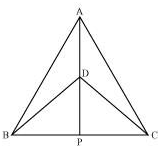(i) In ΔABD and ΔACD,

AB = AC (Given)

BD = CD (Given)

ΔABD ΔACD (By SSS congruence rule)

BAP = CAP …. (1)

(ii) In ΔABP and ΔACP,

AB = AC (Given)

BAP = CAP [From equation (1)]

AP = AP (Common)

ΔABP ΔACP (By SAS congruence rule)

BP = CP (By CPCT) … (2)

(iii) From equation (1),

BAP = CAP

Hence, AP bisects A.

In ΔBDP and ΔCDP,

BD = CD (Given)

DP = DP (Common)

BP = CP [From equation (2)]

ΔBDP ΔCDP (By S.S.S. Congruence rule)

BDP = CDP (By CPCT) … (3)

Hence, AP bisects D.

(iv) ΔBDP ΔCDP

BPD = CPD (By CPCT) …. (4)

BPD + CPD = 180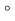(Linear pair angles)

BPD + BPD = 1802BPD = 180[From equation (4)]

BPD = 90… (5)

From equations (2) and (5), it can be saidthat AP is the perpendicular bisector of BC.

Question 2 :

AD is an altitude of an isosceles triangles ABC in whichAB = AC. Show that

Answer 2 :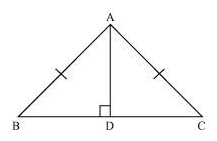AB = AC (Given)

BD = CD (By CPCT)

(ii) Also, by CPCT,

Question 3 :

Two sides AB and BC and median AM of one triangle ABC arerespectively equal to sides PQ and QR and median PN of ΔPQR (see the given figure).Show that:

(i) ΔABM ΔPQN

(ii) ΔABC ΔPQR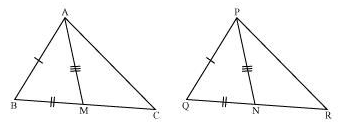(i) In ΔABC, AM is the median to BC.

BM =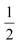BC

In ΔPQR, PN is the median to QR.

QN =QR

However, BC = QRBC =QR

BM = QN … (1)

In ΔABM and ΔPQN,

AB = PQ (Given)

BM = QN [From equation (1)]

AM = PN (Given)

ΔABM ΔPQN (SSS congruence rule)

ABM = PQN (By CPCT)

ABC = PQR … (2)

(ii) In ΔABC and ΔPQR,

AB = PQ (Given)

ABC = PQR [From equation (2)]

BC = QR (Given)

ΔABC ΔPQR (By SAS congruence rule)

Question 4 :

BE and CF are two equal altitudes of atriangle ABC. Using RHS congruence rule, prove that the triangle ABC isisosceles.

Answer 4 :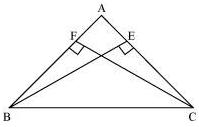In ΔBEC and ΔCFB,

BEC = CFB (Each 90°)

BC = CB (Common)

BE = CF (Given)

ΔBEC ΔCFB (By RHS congruency)

BCE = CBF (By CPCT)

AB = AC (Sides opposite to equal angles of a triangle areequal)

Hence, ΔABC is isosceles.

Question 5 :

ABC is an isosceles triangle with AB = AC.Drawn AP BC to show that B = C.

Answer 5 :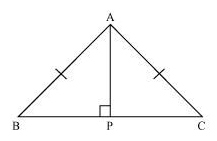In ΔAPB and ΔAPC,

APB = APC (Each 90º)

AB =AC (Given)

AP = AP (Common)

ΔAPB ΔAPC (Using RHS congruence rule)

B = C (By using CPCT)

Todays Deals### Chapter 7 Triangles Ex-7.3 Contributorskrishan

Name:
Email:

# Latest News# 9000 interview questions in different categories